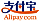Bookcover of Fuzzy Probability Spaces, Fuzzy Dynamical Systems, and Entropy
Booktitle:

# Fuzzy Probability Spaces, Fuzzy Dynamical Systems, and Entropy

## Fuzzy modifications of some concepts of classical probability theory, measure theory, and ergodic theory

LAP LAMBERT Academic Publishing (2013-09-12 )eligible for voucher
ISBN-13:

### 978-3-659-46334-1

ISBN-10:
3659463345
EAN:
9783659463341
Book language:
English
Blurb/Shorttext:
When planning experiments it is very important to know the amount of information that we obtain from their realization. It is known that a measure of information is entropy. A usual mathematical model of experiment in the classical information theory is a measurable partition. Partitions are standardly defined in the context of classical sets. It has appeared however, that for a solution of real problems are partitions defined by means of the concept of fuzzy sets more appropriate. The book contains a review of results of author related to the study of entropy in the fuzzy case. These results represent fuzzy generalizations of some concepts from the classical probability theory. The entropy of fuzzy partition can be considered as a measure of information of experiment, the results of which are fuzzy events. The concept of entropy of a fuzzy partition is exploited to define the entropy of fuzzy dynamical systems. Subsequently an ergodic theory for these systems is proposed. Presented results include the corresponding results from the classical ergodic theory as particular cases. The publication might be useful for specialists who deal in their research work with similar issues.
Publishing house:
Website:
https://www.lap-publishing.com/
By (author) :
Dagmar Markechová
Number of pages:
120
Published on:
2013-09-12
Stock:
Available
Category:
Mathematics
Price:
39.90 €
Keywords:
Entropy, Fuzzy Partition, isomorphism, conjugation, Fuzzy probability space, F-quantum space, Fuzzy dynamical system### Categories